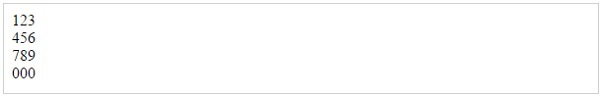# PHP - Function split()

## Syntax

```array split (string pattern, string string [, int limit])
```

## Definition and Usage

The split() function will divide a string into various elements, the boundaries of each element based on the occurrence of pattern in string.

The optional input parameter limit is used to signify the number of elements into which the string should be divided, starting from the left end of the string and working rightward.

In cases where the pattern is an alphabetical character, split() is case sensitive.

## Return Value

• Returns an array of strings after splitting up a string.

## Example

Following is the piece of code, copy and paste this code into a file and verify the result.

```<?php

\$ip = "123.456.789.000"; // some IP address
\$iparr = split ("\.", \$ip);

print "\$iparr <br />";
print "\$iparr <br />" ;
print "\$iparr <br />"  ;
print "\$iparr <br />"  ;

?>
```

This will produce the following result −php_regular_expression.htm